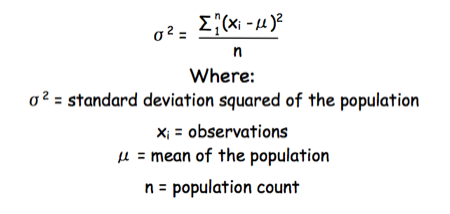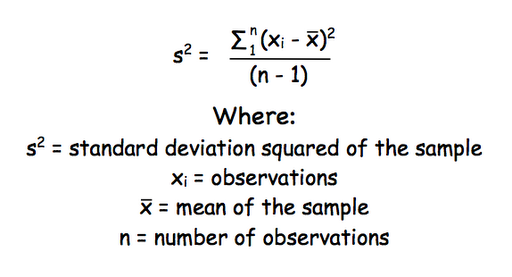## Friday, 11 December 2009

### CMB1000:BGM1001 - why did I get my standard deviation calculation wrong?

A number of the class have asked why (or how) they got the standard deviation calculation wrong in the assessment.

Having looked at the class answers it would appear that the main reason students got it wrong is because they were calculating the standard deviation of a POPULATION and not of a SAMPLE.

The equation for the standard deviation of a population (remember a population is ALL possible examples of a measurement/object and is something that is normally difficult to measure - think of the apple example in the lecture - as all examples can't be collected) is:Equation for the standard deviation of a population

Whereas the standard deviation of a sample (which is what we had) is calculated by:Equation for the standard deviation of a sample

Note that the real differences between the two equation are that in the calculation of the standard deviation of a population we are using the true population mean (µ), whereas in the calculation of the standard deviation of a sample we are using an estimate of the mean (x bar), plus in the calculation of the standard deviation of a sample we are using n - 1 (also called degrees of freedom) as this gives better estimate of the true standard deviation of a population (and we are working with a sample).

#### 1 comment:

1.